# SAT II Math II : Elementary Operations

## Example Questions

### Example Question #1 : Elementary Operations

Add the following numbers: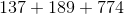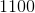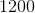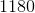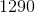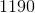Explanation: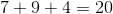Add the tens digits with the two as the carryover.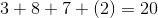Add the hundreds digits with the two as the carryover.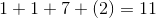Combine this number with the ones digits of the previous calculations.

The answer is:### Example Question #2 : Elementary Operations

Add the numbers: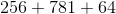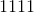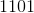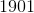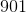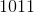Explanation: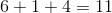Carry the one, and add the tens digits.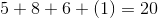Carry the two, and add the hundreds digits.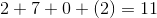Combine this number with the ones digit of the other numbers.

The answer is:### Example Question #3 : Elementary Operations

Add the numbers: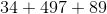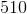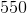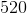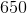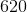Explanation: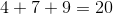Carry the two, and add the tens digits.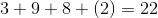Carry the two, and add the hundreds places.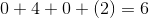Combine all ones digits.

The answer is:### Example Question #4 : Elementary Operations

Add the numbers: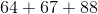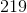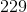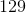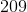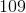Explanation: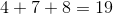Add the tens digits using the one as the carryover.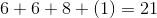Combine this number with the ones digit of the first number solved.

The answer is:### Example Question #5 : Elementary Operations

Add the numbers: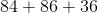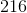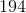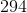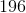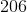Explanation: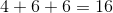Carry the one and add the tens digits.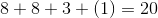Combine this number with the ones digit of the first number.

The answer is:### Example Question #6 : Elementary Operations

Add the following numbers: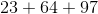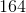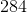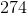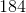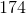Explanation:Carry the one and add the tens digits.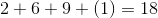Combine this number with the ones digit of the first number.

The answer is:### Example Question #7 : Elementary Operations

Add the numbers: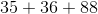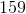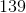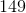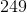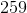Explanation: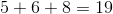Add the tens digits with the one as the carryover.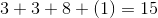The answer is:### Example Question #8 : Elementary Operations

Add the numbers: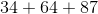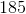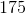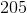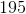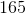Explanation: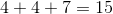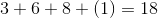The answer is: